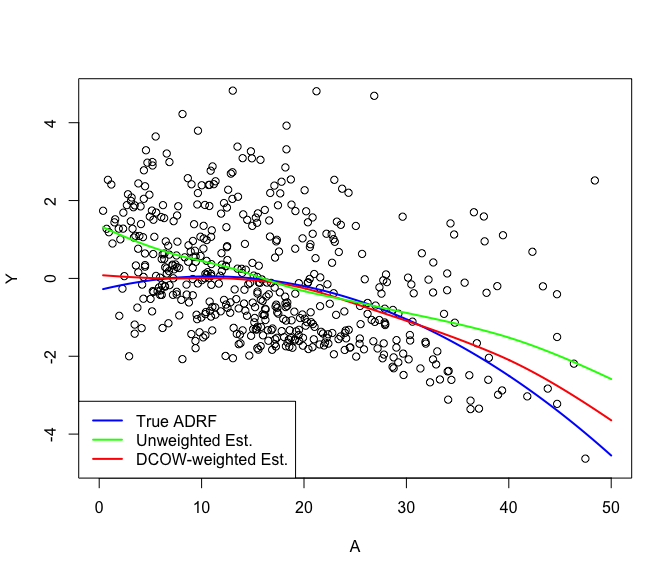# independenceWeights

The `independenceWeights` package constructs weights designed to minimize the weighted statistical dependence between a continuous exposure variable and a vector of confounder variables and implements the methods of Huling, Greifer, and Chen (2021) for doing so. In estimating a causal dose-response function, confounding bias is a function of the dependence between confounders and the exposure/treatment, so weights that minimize the dependence aim to mitigate confounding bias directly.

## Installation

Install the development version from GitHub with:

``````# install.packages("devtools")
devtools::install_github("jaredhuling/independenceWeights")``````

## Example

This is a basic example which shows how to estimate and utilize the distance covariance optimal weights (DCOWs) of Huling, Greifer, and Chen (2021):

``library(independenceWeights)``

Simulate data with a continuous treatment that has a confounded relationship with a response. Data are simulated according to the simulation setup of Vegetabile et al. (2021).

``````simdat <- simulate_confounded_data(seed = 999, nobs = 500)
y <- simdat\$data\$Y ## response
A <- simdat\$data\$A ## treatment
X <- as.matrix(simdat\$data[c("Z1", "Z2", "Z3", "Z4", "Z5")]) ## confounders``````

Now estimate weights to adjust for confounders using the distance covariance optimal weights (DCOWs), which aim to mitigate the dependence between A and X:

``````dcows <- independence_weights(A, X)

dcows
#> Unweighted distance covariance:           0.3963
#> Optimized weighted dependence distance:   0.0246
#> Effective sample size:                  264.0099
#>
#> Weight ranges:
#>    Min. 1st Qu.  Median    Mean 3rd Qu.    Max.
#>  0.0000  0.2767  0.8215  1.0000  1.4120  5.7360``````

Alternatively, information about any set of weights can be printed via `weighted_energy_stats()`. The unweighted distance covariance is a measure of the unadjusted dependence between confounders and treatment. The weighted dependence distance is a measure of this dependence after weighting. The weighted energy distance between A and weighted A measures how well the marginal distribution of A is preserved after weighting. Similarly for X. The effective sample size estimates the effective sample size after weighting.

``````weighted_energy_stats(A, X, dcows\$weights)
#> Unweighted distance covariance:            0.3963
#> Weighted dependence distance:              0.0246
#> Weighted energy distance(A, weighted A):   0.0014
#> Weighted energy distance(X, weighted X):   0.0025
#> Effective sample size:                   264.0099``````

We can assess traditional measures of balance such as marginal weighted correlations with `cobalt`:

``library(cobalt)``

Even though the DCOWs aim to mitigate joint dependence between X and A, they result in very small marginal weighted correlations between them as well. (changing `poly` to 10 below would reveal that even up to 10th degree polynomials of covariates as well-decorrelated):

``````bal.tab(A ~ Z1 + Z2 + Z3 + Z4 + Z5, data = data.frame(A = A, X),
weights = list(DCOW = dcows\$weights),
un = TRUE, int = TRUE, poly = 1, stats = "cor")
#> Balance Measures
#>              Type Corr.Un Corr.Adj
#> Z1        Contin. -0.1351  -0.0021
#> Z2        Contin.  0.4445   0.0161
#> Z3        Contin. -0.0397  -0.0284
#> Z4        Contin.  0.0267  -0.0057
#> Z5         Binary  0.0691   0.0009
#> Z1 * Z2   Contin. -0.0944   0.0016
#> Z1 * Z3   Contin. -0.1539  -0.0080
#> Z1 * Z4   Contin. -0.0168  -0.0007
#> Z1 * Z5_0 Contin. -0.1445  -0.0101
#> Z1 * Z5_1 Contin.  0.0438   0.0101
#> Z2 * Z3   Contin.  0.2647  -0.0129
#> Z2 * Z4   Contin.  0.0488  -0.0027
#> Z2 * Z5_0 Contin. -0.0266  -0.0005
#> Z2 * Z5_1 Contin.  0.0884   0.0027
#> Z3 * Z4   Contin.  0.0260  -0.0077
#> Z3 * Z5_0 Contin. -0.0730  -0.0064
#> Z3 * Z5_1 Contin.  0.0669   0.0019
#> Z4 * Z5_0 Contin. -0.0016  -0.0038
#> Z4 * Z5_1 Contin.  0.0486  -0.0037
#>
#> Effective sample sizes
#>             Total

We can also minimize the weighted dependence subject to the constraint that marginal moments of X and A are decorrelated:

``````dcows_decor <- independence_weights(A, X,
decorrelate_moments = TRUE)

dcows_decor
#> Unweighted distance covariance:           0.3963
#> Optimized weighted dependence distance:   0.0251
#> Effective sample size:                  260.8907
#>
#> Weight ranges:
#>    Min. 1st Qu.  Median    Mean 3rd Qu.    Max.
#>  0.0000  0.2491  0.8344  1.0000  1.4330  5.7290``````
``````bal.tab(A ~ Z1 + Z2 + Z3 + Z4 + Z5, data = data.frame(A = A, X),
weights = list(DCOW = dcows\$weights,
DCOW_decor = dcows_decor\$weights),
un = TRUE, int = TRUE, stats = "cor")
#> Balance Measures
#>              Type Corr.Un Corr.DCOW Corr.DCOW_decor
#> Z1        Contin. -0.1351   -0.0021          0.0000
#> Z2        Contin.  0.4445    0.0161         -0.0004
#> Z3        Contin. -0.0397   -0.0284         -0.0002
#> Z4        Contin.  0.0267   -0.0057         -0.0002
#> Z5         Binary  0.0691    0.0009         -0.0000
#> Z1 * Z2   Contin. -0.0944    0.0016          0.0023
#> Z1 * Z3   Contin. -0.1539   -0.0080         -0.0021
#> Z1 * Z4   Contin. -0.0168   -0.0007          0.0058
#> Z1 * Z5_0 Contin. -0.1445   -0.0101         -0.0087
#> Z1 * Z5_1 Contin.  0.0438    0.0101          0.0104
#> Z2 * Z3   Contin.  0.2647   -0.0129         -0.0024
#> Z2 * Z4   Contin.  0.0488   -0.0027          0.0019
#> Z2 * Z5_0 Contin. -0.0266   -0.0005         -0.0012
#> Z2 * Z5_1 Contin.  0.0884    0.0027          0.0012
#> Z3 * Z4   Contin.  0.0260   -0.0077         -0.0011
#> Z3 * Z5_0 Contin. -0.0730   -0.0064         -0.0020
#> Z3 * Z5_1 Contin.  0.0669    0.0019          0.0019
#> Z4 * Z5_0 Contin. -0.0016   -0.0038          0.0018
#> Z4 * Z5_1 Contin.  0.0486   -0.0037         -0.0032
#>
#> Effective sample sizes
#>             Total
#> All        500.
#> DCOW       264.01
#> DCOW_decor 260.89``````

Now use the weights to estimate the causal average dose response function (ADRF)

``````## create grid
trt_vec <- seq(min(simdat\$data\$A), 50, length.out=500)

adrf_hat <- weighted_kernel_est(A, y, dcows\$weights, trt_vec)\$est

## estimate naively without weights
adrf_hat_unwtd <- weighted_kernel_est(A, y, rep(1, length(y)), trt_vec)\$est

ylims <- c(-4.75, 4.75)
plot(x = simdat\$data\$A, y = simdat\$data\$Y, ylim = ylims,
xlim = c(0,50),
xlab = "A", ylab = "Y")